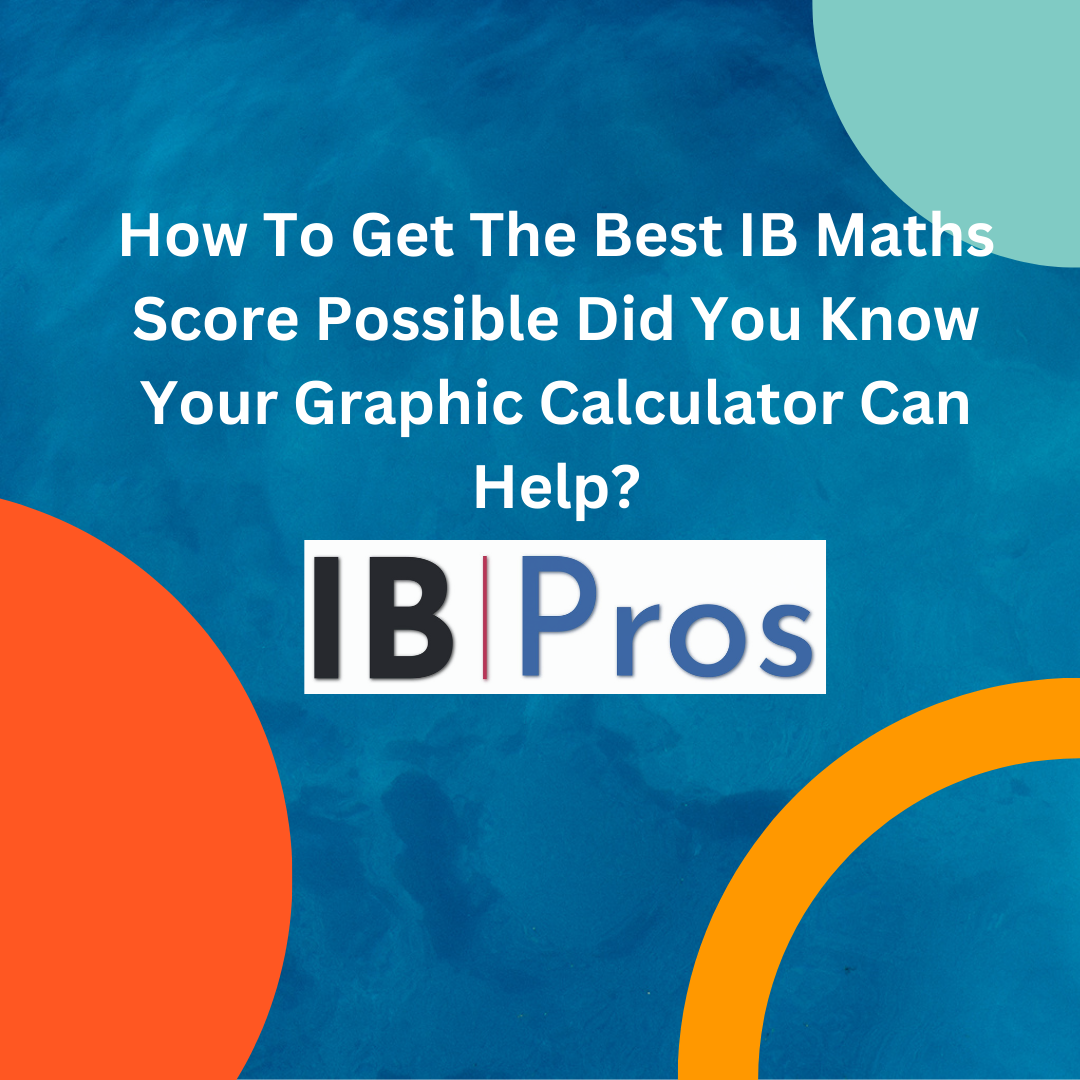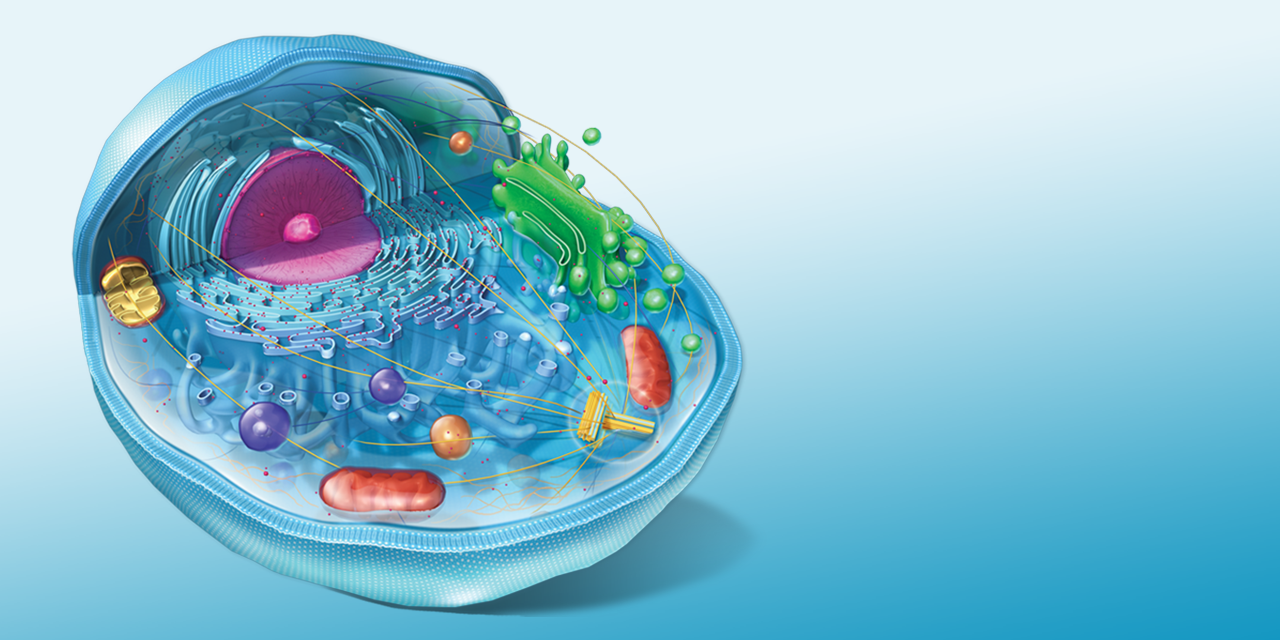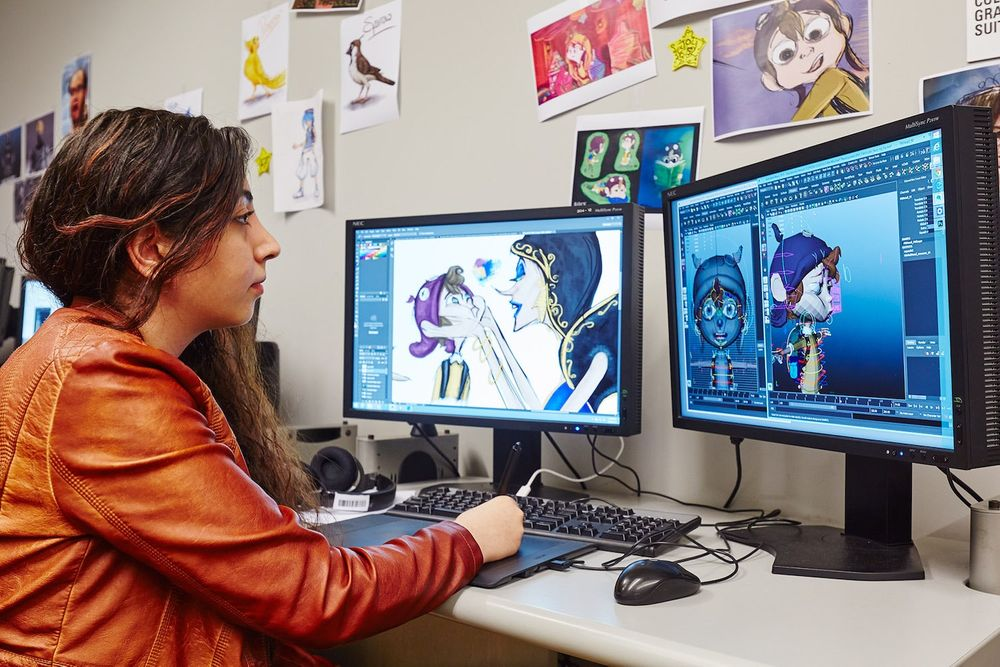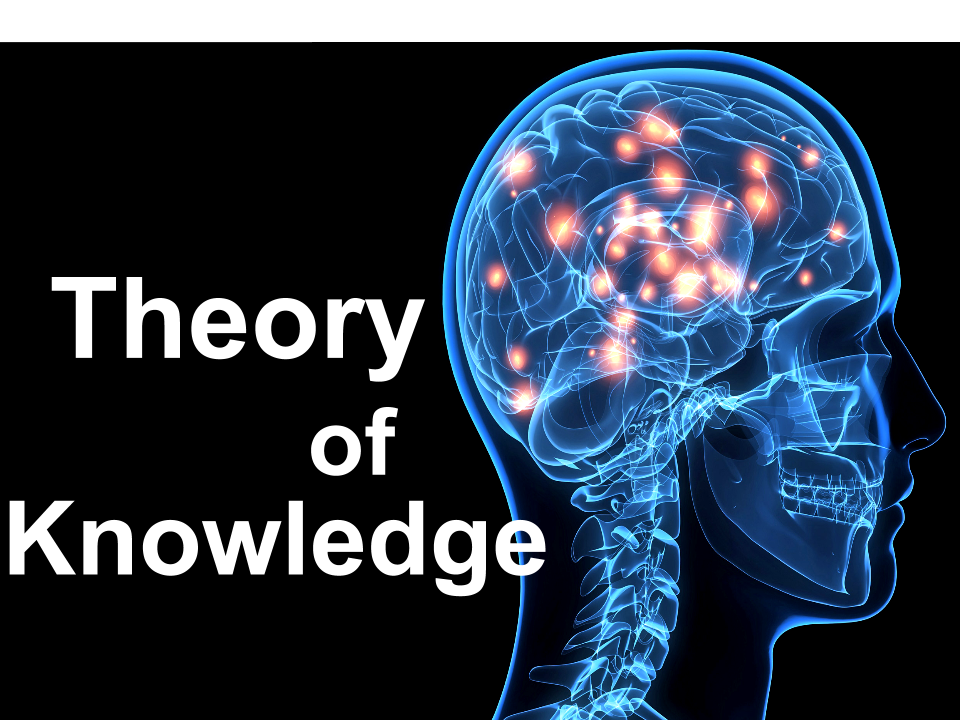# Explore Our Subjects & Hire Your Private Tutor

We cover all subjects in IB fo DP1, DP2 and MYPMathematics

### MathematicsPhysics

### PhysicsChemistry

### ChemistryArabic

### ArabicEnglish

### EnglishBiology

### BiologyEconomics

### EconomicsSpanish

### SpanishFrench

### FrenchVisual Arts

### Visual ArtsGlobal Politics

### Global PoliticsUniversity Essay

### University EssayExtended Essays

### Extended EssaysESS

### ESSPhilosophy

### PhilosophyComputer Science

### Computer ScienceGeography

### GeographyCreativity, Activity, Service

### Creativity, Activity, ServicePsychology

### PsychologyHistory

### HistoryTheory of Knowledge

### Theory of Knowledge

IB Math

In the pursuit of achieving the best score in IB Maths, students often overlook the potential benefits of utilizing a graphic calculator. This powerful tool can greatly enhance problem-solving efficiency and aid in obtaining optimal results.

By adjusting the calculator's settings for trigonometry and becoming familiar with its various features, students can unlock its full potential. From finding correlation coefficients to solving equations, the graphic calculator offers a wide range of functions that can streamline mathematical processes.

Additionally, it can be used for tasks such as working with sequences and series, verifying calculations, and minimizing algebraic manipulations to save valuable time. However, it is crucial to activate the calculator early on in problem-solving and to ensure compliance with IB regulations by deleting any unauthorized programs.

Furthermore, it is advisable to contact IB Pros for tuition with a qualified teacher and access additional articles on this subject. With a fully charged graphic calculator in hand, students can unlock their mathematical potential and strive for their best possible score in IB Maths.

## Key Takeaways

• Adjusting calculator settings for trigonometry and becoming familiar with its features is important.
• Utilizing the features of a graphic calculator effectively can improve problem-solving efficiency in IB Maths.
• Activating the calculator early in problem-solving saves time.
• Minimizing algebra and using the calculator for calculations simplifies complex equations.

## How to optimize calculator settings

One important aspect of maximizing your IB Maths score is optimizing the settings on your graphic calculator. This includes ensuring that the calculator is in the correct mode for Trigonometry, specifically in radians.

Familiarizing yourself with adjusting window settings on the calculator is also crucial.

Knowing how to find the correlation coefficient (r) and utilizing apps on the calculator to solve simultaneous equations and find polynomial roots can significantly enhance your score.

Additionally, utilizing the table function for sequences and series and understanding how the calculator displays asymptotes and graph features can be beneficial.

While the calculator can be used to check calculations, it is important to provide evidence of the calculation process. Showing sketch graphs, table portions, and lines of working when using the calculator is advised.

Minimizing algebra and utilizing the calculator to save time is another useful strategy.

Activating the calculator as soon as possible in problem-solving and deleting unpermitted programs on the calculator are also important factors to consider.

Finally, ensuring that the calculator is fully charged and having extra batteries is essential.

## Tips for problem-solving efficiency

To improve problem-solving efficiency in IB Maths, it is important to utilize the features of a graphic calculator effectively.

Firstly, activate the calculator as soon as possible in problem-solving to save time.

Secondly, minimize algebra and use the calculator to perform calculations, which helps in simplifying complex equations.

Lastly, make use of apps on the calculator to solve simultaneous equations and find polynomial roots, as this can significantly speed up the problem-solving process.

By following these tips, students can effectively use their graphic calculators to optimize their problem-solving efficiency and improve their overall performance in IB Maths.

## Utilizing calculator functions effectively

Utilizing the various functions of a graphic calculator effectively can greatly enhance problem-solving efficiency in IB Maths. A graphic calculator offers a range of tools that can simplify complex calculations and provide visual representations of mathematical concepts.

One important feature is the ability to adjust the calculator's mode for trigonometry, ensuring that calculations involving angles are done in radians, which aligns with the IB Maths curriculum.

Additionally, knowing how to manipulate the calculator's window settings allows for a better understanding of graph features and asymptotes.

The calculator's apps can be used to solve simultaneous equations and find polynomial roots, saving time and minimizing the need for extensive algebraic calculations.

The table function is also valuable for working with sequences and series.

By activating the calculator early in problem-solving and using it to check calculations, students can provide evidence of their work and ensure accuracy.

Overall, effectively utilizing a graphic calculator can be a powerful tool in achieving the best possible IB Maths score.

## Conclusion

The article 'How to Get the Best IB Maths Score Possible: Did You Know Your Graphic Calculator Can Help?' provides valuable tips and strategies for maximizing your score in IB Maths using a graphic calculator.

It suggests adjusting the calculator's settings for trigonometry and becoming familiar with its features, such as finding correlation coefficients and solving equations. By utilizing the calculator effectively, you can save time, check calculations, and minimize algebra.

It is important to activate the calculator early in problem-solving and delete any unpermitted programs. Ensure the calculator is fully charged and allowed by the IB.

For additional support, consider contacting IB Pros for tuition with a qualified teacher and accessing more informative articles on the subject.